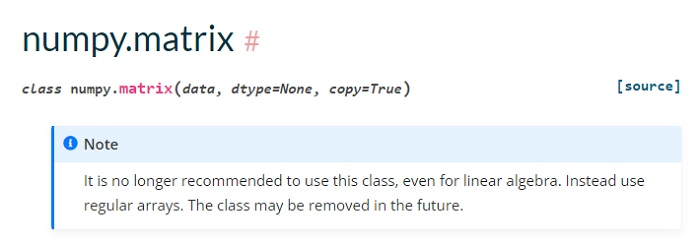# Difference Between Matrices and Arrays in Python?

The arrays in Python are ndarray objects. The matrix objects are strictly 2-dimensional whereas the ndarray objects can be multi-dimensional. To create arrays in Python, use the Numpy library.

## Matrices in Python

Matrix is a special case of two-dimensional array where each data element is of strictly same size. Matrices are a key data structure for many mathematical and scientific calculations. Every matrix is also a two-dimensional array but not vice versa. Matrix objects are a subclass of ndarray, so they inherit all the attributes and methods of ndarrays.

### Example

Create a Matrix and Display

from numpy import *

# Create a Matrix
mat = array([['A',12,16],['B',11,13],
['C',20,19],['D',22,21],
['E',18,22],['F',12,18]])

# Display the Matrix
print("Matrix = \n",mat)


### Output

Matrix =
[['A' '12' '16']
['B' '11' '13']
['C' '20' '19']
['D' '22' '21']
['E' '18' '22']
['F' '12' '18']]


### Example

Create a Matrix with mat()

The mat() function interprets an input as matrix -

import numpy as np

# Create a Matrix
mat = np.mat([[5,10],[15,20]])

# Display the Matrix
print("Matrix = \n",mat)


### Output

Matrix =
[[ 5 10]
[15 20]]


## Arrays in Python

Array is a container which can hold a fix number of items and these items should be of the same type. To work with arrays in Python, import the NumPy library.

### Example

import numpy as np

# Create an array
arr = np.array([5, 10, 15, 20])

# Display the Array
print("Array =\n")
for x in arr:
print(x)


### Output

Array =

5
10
15
20


## Matrix Operations with Numpy Arrays

As per the official documentation, the class numpy.matrix will be removed in the future. Therefore, now the matrix algebra operations has to be done with Numpy Arrays.### Example

Let us now see some matrix operators with Numpy Arrays. First, we will create a matrix using arrays -

import numpy as np

# Create Matrices
mat1 = np.array([[5,10],[3,9]])
mat2 = np.array([[15,20],[10,11]])

# Display the Matrices
print("Matrix1 = \n",mat1)
print("Matrix2 = \n",mat2)


### Output

Matrix1 =
[[ 5 10]
[ 3  9]]
Matrix2 =
[[15 20]
[10 11]]


### Example

Let us multiply the above matrices -

import numpy as np

# Create Matrices
mat1 = np.array([[5,10],[3,9]])
mat2 = np.array([[15,20],[10,11]])

# Display the Matrices
print("Matrix1 = \n",mat1)
print("Matrix2 = \n",mat2)
mulMat = mat1@mat2
print("\nMatrix Multiplication = \n",mulMat)


### Output

Matrix1 =
[[ 5 10]
[ 3  9]]
Matrix2 =
[[15 20]
[10 11]]

Matrix Multiplication =
[[175 210]
[135 159]]


### Example

Get the Transpose -

import numpy as np

# Create Matrices
mat1 = np.array([[5,10],[3,9]])
mat2 = np.array([[15,20],[10,11]])

# Display the Matrices
print("Matrix1 = \n",mat1)
print("Matrix2 = \n",mat2)
mulMat = mat1@mat2
print("\nTranspose = \n",mulMat.T)


### Output

Matrix1 =
[[ 5 10]
[ 3  9]]
Matrix2 =
[[15 20]
[10 11]]

Transpose =
[[175 135]
[210 159]]